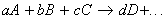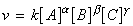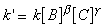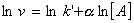# Describe isolation method in determination of rate law & order of reaction

Dear Student,

The tedious step in reaction mixtures is determining its kinetic mechanism (rate law and order) which are performed using the best method of Isolation techniques. The isolation technique is proceeded with reducing the concentration of reactant to the smaller than when comparing with other reactant, by using this all the concentrations with zero,first and second order kinetics plot are interpreted using concentration-time data except the reactant with constant conditions.

For example the conversion of methyl bromide to methanol using substitution reaction is expressed below for quick grasping of the concept,

The slope and intercept values are used in determination of the isolation rate of the molecules, by using the interpreted data (concentration) the order of reaction are expressed below,

Regards.

• 2
The easiest method to determine a rate law is by the isolation method where the concentrations of all the reactants except one is in large excess. Thus for example, if for the reaction:which has a rate law of the form:we let B and C to be in large excess with concentrations [Bo] and [Co], then it may be assumed that their concentration is constant throughout the reaction. Thus we may write:where:This means that if we take logs of both sides we get:which is the equation of a straight line:for plotting ln(v) against ln[A] with gradient a and y-intercept of ln(k`).

Note that:

• Under these conditions, we may say that the reaction has a pseudo -order rather than the true-order.
• The process can be repeated by letting A/C and then A/B be in excess to determine a, b, g, and k.

In this method all the reactants are taken in very large excess except one and the order of the reaction is determined by the first method. The concentrations of the substances taken in excess are considered to be constant. The sum of the orders, obtained by the process of isolation of each reactant is the order of the reaction. The method may be illustrated by the reaction between sodium formate and silver acetate.

When silver acetate is taken in large excess, then the reaction is of first order, but when sodium formate is taken in excess the reaction is of second order. Hence the reaction is of third order.

regards

• 1
What are you looking for?